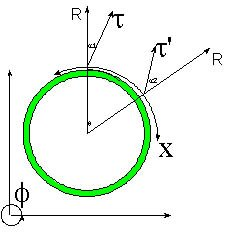# Hypergeometrical Universe

## The Meaning of Planck's ConstantThe Meaning of Planck's Constant

Among all Cosmological Constants, Planck's constant has a preeminent position. It is the basis of anything that is Quantized. From Black Body Radiation to the Quantization of Light to the Quantization of Action and thus Quantum Mechanics.

In my theory, it has a new meaning.

My theory is a purely geometrical theory without any parameters. Planck's constant is used to relate the fundamental wavelength (de Broglie Hyperspherical Universe Expansion Wavelength) to the mass of a Fat Electron. That might seem like I am using a parameter...:)

In fact, Science has been using this parameter to relate the Fat Electron 3D Volume Projection to its mass using equivalent momentum analysis (h=m.v.lambda). The parametrization happened when one tries to match the resulting de Broglie wavelength to the 3D de Broglie projections of the associated 4D dilatons that electrons surfed in their 4D trajectory.

The reasoning goes like this: Monochromatic electrons traveling along a 3D trajectory Electrons surf a 4D Dilaton. The 5D dilaton is recognized as the 3D de Broglie matter wave associated incorrectly to a single Electron as opposed to being an ensemble property. To match that 3D projected wavelength to the wavelenght associated with the Skinny Electron mass one needs the Planck's constant.

The same reasoning can be done when one tries to model Black Body Radiation and has to deal with a dilaton bath 3D projection.

In conclusion: The 4D dilaton has a wavelength that does not depend upon Mass. Its 3D projection is the 3D de Broglie Matter waves. The momentum equation used to match 3D de Broglie wavelenght to the particle mass has a Planck's Constant as a proportionality constant. This means that Planck constant was originally a relationship between 4D volumes and 3D Masses.

Cheers,

MP
Currently unrated

or

RSS / Atom